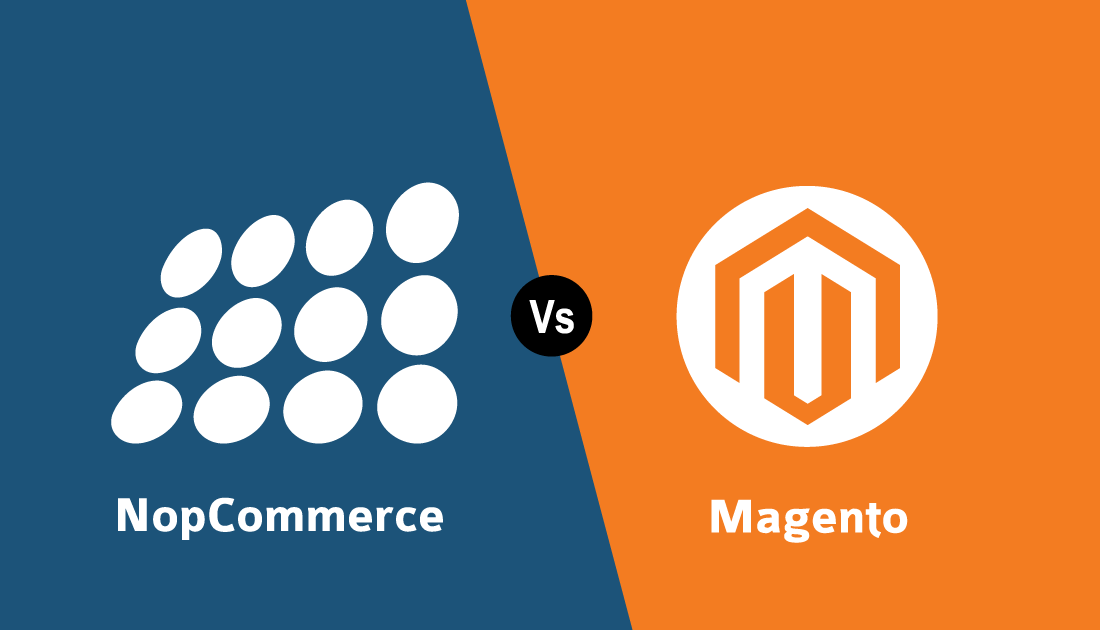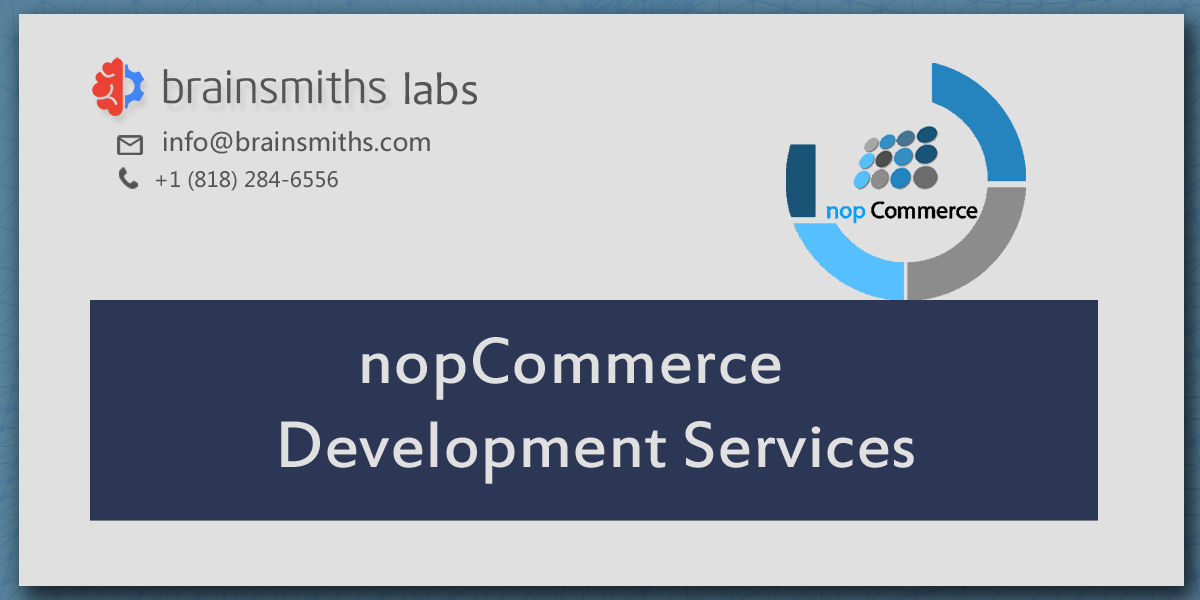What Brainsmiths Labs can do for you ?

## Python Training

Brainsmiths Labs’s comprehensive Python Training Course will teach you the basics of Python, data operations, conditional statements, shell scripting, and Django. This course will give you hands-on development experience and prepare you for an exciting career as a professional Python programmer.

### Curriculum

Learning Objectives: You will get a brief idea of what Python is and touch on the basics.

Topics:

• Overview of Python
• The Companies using Python
• Different Applications where Python is used
• Discuss Python Scripts on UNIX/Windows
• Values, Types, Variables
• Operands and Expressions
• Conditional Statements
• Loops
• Command Line Arguments
• Writing to the screen

Hands On/Demo:

• Creating “Hello World” code
• Variables
• Demonstrating Conditional Statements
• Demonstrating Loops

Skills:

• Fundamentals of Python programming

Learning Objectives: Learn different types of sequence structures, related operations and their usage. Also learn diverse ways of opening, reading, and writing to files.

Topics:

• Python files I/O Functions
• Numbers
• Strings and related operations
• Tuples and related operations
• Lists and related operations
• Dictionaries and related operations
• Sets and related operations

Hands On/Demo:

• Tuple - properties, related operations, compared with a list
• List - properties, related operations
• Dictionary - properties, related operations
• Set - properties, related operations

Skills:

• File Operations using Python
• Working with data types of Python

Learning Objectives: In this Module, you will learn how to create generic python scripts, how to address errors/exceptions in code and finally how to extract/filter content using regex.

Topics:

• Functions
• Function Parameters
• Global Variables
• Variable Scope and Returning Values
• Lambda Functions
• Object-Oriented Concepts
• Standard Libraries
• Modules Used in Python
• The Import Statements
• Module Search Path
• Package Installation Ways
• Errors and Exception Handling
• Handling Multiple Exceptions

Hands On/Demo:

• Functions - Syntax, Arguments, Keyword Arguments, Return Values
• Lambda - Features, Syntax, Options, Compared with the Functions
• Sorting - Sequences, Dictionaries, Limitations of Sorting
• Errors and Exceptions - Types of Issues, Remediation
• Packages and Module - Modules, Import Options, sys Path

Skills:

• Error and Exception management in Python
• Working with functions in Python

Learning Objectives: This Module helps you get familiar with basics of statistics, different types of measures and probability distributions, and the supporting libraries in Python that assist in these operations. Also, you will learn in detail about data visualization.

Topics:

• NumPy - arrays
• Operations on arrays
• Indexing slicing and iterating
• Reading and writing arrays on files
• Pandas - data structures & index operations
• Reading and Writing data from Excel/CSV formats into Pandas
• matplotlib library
• Grids, axes, plots
• Markers, colours, fonts and styling
• Types of plots - bar graphs, pie charts, histograms
• Contour plots

Hands On/Demo:

• NumPy library- Creating NumPy array, operations performed on NumPy array
• Pandas library- Creating series and dataframes, Importing and exporting data
• Matplotlib - Using Scatterplot, histogram, bar graph, pie chart to show information, Styling of Plot

Skills:

• Probability Distributions in Python
• Python for Data Visualization

Learning Objectives: Through this Module, you will understand in detail about Data Manipulation

Topics:

• Basic Functionalities of a data object
• Merging of Data objects
• Concatenation of data objects
• Types of Joins on data objects
• Exploring a Dataset
• Analysing a dataset

Hands On/Demo:

• Pandas Function- Ndim(), axes(), values(), head(), tail(), sum(), std(), iteritems(), iterrows(), itertuples()
• GroupBy operations
• Aggregation
• Concatenation
• Merging
• Joining

Skills:

• Python in Data Manipulation

Learning Objectives: In this module, you will learn the concept of Machine Learning and its types.

Topics:

• Python Revision (numpy, Pandas, scikit learn, matplotlib)
• What is Machine Learning?
• Machine Learning Use-Cases
• Machine Learning Process Flow
• Machine Learning Categories
• Linear regression

Hands On/Demo:

• Linear Regression – Boston Dataset

Skills:

• Machine Learning concepts
• Machine Learning types
• Linear Regression Implementation

Learning Objectives: In this module, you will learn Supervised Learning Techniques and their implementation, for example, Decision Trees, Random Forest Classifier etc.

Topics:

• What are Classification and its use cases?
• What is Decision Tree?
• Algorithm for Decision Tree Induction
• Creating a Perfect Decision Tree
• Confusion Matrix
• What is Random Forest?

Hands On/Demo:

• Implementation of Logistic regression
• Decision tree
• Random forest

Skills:

• Supervised Learning concepts
• Implementing different types of Supervised Learning algorithms
• Evaluating model output

Learning Objectives: In this module, you will learn about the impact of dimensions within data. You will be taught to perform factor analysis using PCA and compress dimensions. Also, you will be developing LDA model.

Topics:

• Introduction to Dimensionality
• Why Dimensionality Reduction
• PCA
• Factor Analysis
• Scaling dimensional model
• LDA

Hands On/Demo:

• PCA
• Scaling

Skills:

• Implementing Dimensionality Reduction Technique

Learning Objectives: In this module, you will learn about Unsupervised Learning and the various types of clustering that can be used to analyze the data.

Topics:

• What is Clustering & its Use Cases?
• What is K-means Clustering?
• How does K-means algorithm work?
• How to do optimal clustering
• What is C-means Clustering?
• What is Hierarchical Clustering?
• How Hierarchical Clustering works?

Hands On/Demo:

• Implementing K-means Clustering
• Implementing Hierarchical Clustering

Skills:

• Unsupervised Learning
• Implementation of Clustering – various types

Learning Objectives: In this module, you will learn Association rules and their extension towards recommendation engines with Apriori algorithm.

Topics:

• What are Association Rules?
• Association Rule Parameters
• Calculating Association Rule Parameters
• Recommendation Engines
• How does Recommendation Engines work?
• Collaborative Filtering
• Content-Based Filtering

Hands On/Demo:

• Apriori Algorithm

Skills:

• Data Mining using python
• Recommender Systems using python

Learning Objectives: In this module, you will learn about developing a smart learning algorithm such that the learning becomes more and more accurate as time passes by. You will be able to define an optimal solution for an agent based on agent-environment interaction.

Topics:

• What is Reinforcement Learning
• Why Reinforcement Learning
• Elements of Reinforcement Learning
• Exploration vs Exploitation dilemma
• Epsilon Greedy Algorithm
• Markov Decision Process (MDP)
• Q values and V values
• Q – Learning
• α values

Hands On/Demo:

• Calculating Reward
• Discounted Reward
• Calculating Optimal quantities
• Implementing Q Learning
• Setting up an Optimal Action

Skills:

• Implement Reinforcement Learning using python
• Developing Q Learning model in python

Learning Objectives: In this module, you will learn about Time Series Analysis to forecast dependent variables based on time. You will be taught different models for time series modeling such that you analyze a real time-dependent data for forecasting.

Topics:

• What is Time Series Analysis?
• Importance of TSA
• Components of TSA
• White Noise
• AR model
• MA model
• ARMA model
• ARIMA model
• Stationarity
• ACF & PACF

Hands On/Demo:

• Checking Stationarity
• Converting a non-stationary data to stationary
• Implementing Dickey-Fuller Test
• Plot ACF and PACF
• Generating the ARIMA plot
• TSA Forecasting

Skills:

• TSA in Python

Learning Objectives: In this module, you will learn about selecting one model over another. Also, you will learn about Boosting and its importance in Machine Learning. You will learn on how to convert weaker algorithms into stronger ones.

Topics:

• What is Model Selection?
• The need for Model Selection
• Cross-Validation
• What is Boosting?
• How Boosting Algorithms work?
• Types of Boosting Algorithms

Hands On/Demo:

• Cross-Validation

Skills:

• Model Selection
• Boosting algorithm using python

Python is an open-source functional and object-oriented programming language that is popular for its simplicity and extensive library. It is used for building GUI applications, interactive websites and applications, data analysis, artificial intelligence, automation, and much more.

You can enroll at our website and make an online payment using any of the following options:

• Visa credit or debit card
• MasterCard
• PayPal
• Stripe

Once payment is received, you will automatically receive a payment receipt and access information via email.

Payments can be made using any of the following options and a receipt of the same will be issued to you automatically via email.

• Visa credit or debit card
• MasterCard
• PayPal
• Stripe

All of the classes are conducted live online. They are interactive sessions that enable you to ask questions and participate in discussions during class time. We do, however, provide recordings of each session you attend for your future reference.

Brainsmiths Labs provides the course completion certificate once you successfully complete the Certified iOS App Developer training program.

+1 (818) 284-6556

### Top Blogs#### nopcommerce VS Magento